# How to Work with Data Frames in R (8 Examples)

In this tutorial, I’ll explain how to work with data frames in the R programming language.

Let’s get started!

## What is a Data Frame?

Data frames are data structures that are used to store data sets in the R programming language.

More technically, data frames are lists of vectors of equal length and can contain different variable types and classes.

Data frames are very common and probably the most often used data structure in the R programming language.

In case you want to learn more on the definition of data frames, please have a look here.

However, you are here for the R programming example codes. So without too much introductory talk, let’s move right on to the R syntax!

## Example 1: Load Built-In Data Frame

There are basically two ways to import a data frame into the R programming language. Either you may read a data frame from an external file (such as a CSV, a TXT, or an XLSX Excel file), or you may load an already built in data set.

For the sake of simplicity, let’s just import a data frame that is already provided by the basic installation of the R programming language.

The following R code loads the mtcars data set into our current R session. All we have to do is to call the data() function, and we have to specify the name of the data set we want to use (i.e. mtcars).

`data(mtcars)                                    # Load data frame`

After executing the previous line of code, the mtcars data frame is loaded.

We may now use the head() function to print the first six rows of the mtcars data frame to the RStudio console:

`head(mtcars)                                    # Print first six rows of data frame`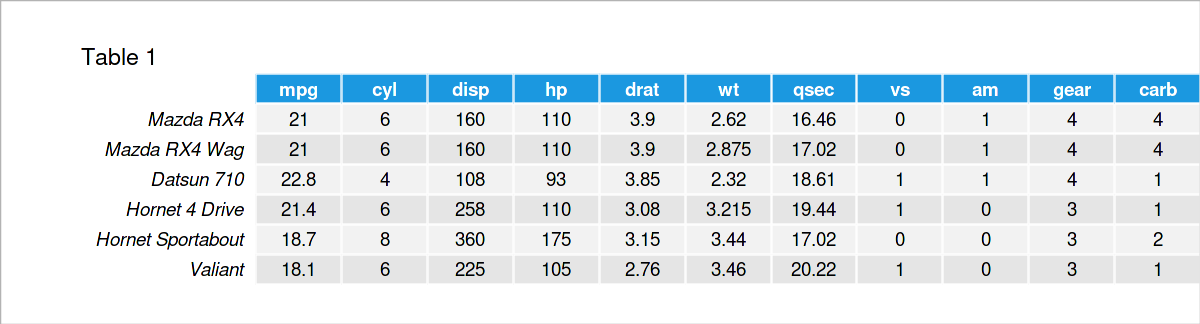As shown in Table 1, the previous code has printed the head of the mtcars data set. It shows the column and row names as well as the values that are stored within the data.

## Example 2: Create User-Defined Data Frame

Alternatively to the import of a data set, we may create our own user-defined data frame.

For this task, we can apply the data.frame() function as shown below. Within the data.frame function, we have to specify the column names and the corresponding values that should be stored within the data:

```data <- data.frame(x1 = c(1, 3, 7, 7, 4, 7),    # Construct data frame manually
x2 = c("a", "x", "b", "x", "x", "c"),
x3 = 10:15)
data                                            # Print manually constructed data frame```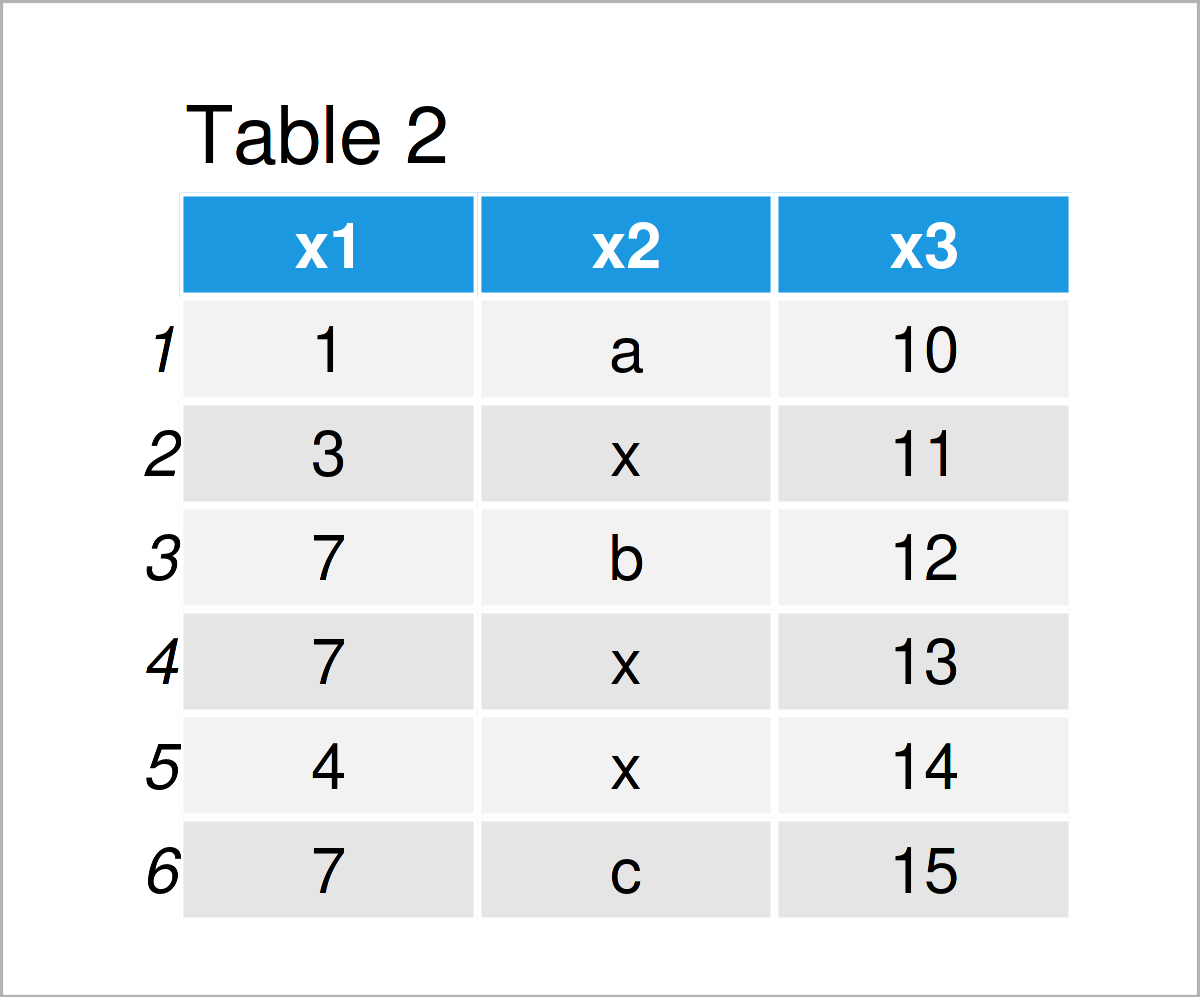In Table 2 you can see that we have created a data frame with six rows and the three variables x1, x2, and x3.

## Example 3: Inspect Data Frame

When you are working with data frames, a very first step is to explore your data. In the following section, we’ll walk through different data exploration steps that can be applied to basically every data frame.

Let’s first have a look at the dimensions of our data. We can get the number of rows using the nrow() function

```nrow(data)                                      # Number of rows
#  6```
```ncol(data)                                      # Number of columns
#  3```

Based on the previous outputs we have already seen that our data has six rows and three columns. However, we can get this result even quicker by simply applying the dim() function.

The first output value of the dim function corresponds to the number of rows and the second output value corresponds to the number of columns:

```dim(data)                                       # Dimensions of data frame
#  6 3```

To get even more information by using only one function, we can apply the the str() function to our data:

```str(data)                                       # Display structure of data frame
# 'data.frame':	6 obs. of  3 variables:
#  \$ x1: num  1 3 7 7 4 7
#  \$ x2: chr  "a" "x" "b" "x" ...
#  \$ x3: int  10 11 12 13 14 15```

The previous output of the str() function once again shows the number of rows and columns. In addition, it returns the data classes of each column and the values that are contained in these columns.

If we are only interested in the data classes of our variables, we may alternatively use the sapply and class functions as shown below.

As you can see, our first column x1 has the numeric class, the second column x2 has the character class, and the third column x3 has the integer class:

```sapply(data, class)                             # Classes of data frame columns
#          x1          x2          x3
#   "numeric" "character"   "integer"```

Let’s move one step further to the analysis of our data frame. The summary() function provides a good overview on some of the most important descriptive statistics:

```summary(data)                                   # Summary statistics of data frame
#        x1             x2                  x3
#  Min.   :1.000   Length:6           Min.   :10.00
#  1st Qu.:3.250   Class :character   1st Qu.:11.25
#  Median :5.500   Mode  :character   Median :12.50
#  Mean   :4.833                      Mean   :12.50
#  3rd Qu.:7.000                      3rd Qu.:13.75
#  Max.   :7.000                      Max.   :15.00```

The output above shows statistical measures such as the minimum value, 1st quartile, median, mean, 3rd quartile, and the maximum value for the numeric/integer variables, as well as the length and the class of character variables.

So far, so good! However, in the previous section I have only explained how to evaluate and explore an entire data set. In the next section, I’ll demonstrate how to extract only certain components of a data frame.

## Example 4: Extract Components of Data Frame

The code below shows how to select only one variable from a data frame.

For this task, we have basically three options. First, we can use the \$ operator:

```data\$x2                                         # Using \$ operator
#  "a" "x" "b" "x" "x" "c"```

As you can see, the previous R code returned only the values of the second column x2 as a vector object.

Alternatively to the previous code, we may use square brackets. Note the comma within the square brackets.

The syntax in front of the comma corresponds to the rows of our data frame, and the syntax after the comma corresponds to the columns.

In this specific example, we want to extract all the rows and only the second column from our data. Hence, we specify the index position 2 on the right side of the comma within the square brackets:

```data[ , 2]                                      # Using [ , ]
#  "a" "x" "b" "x" "x" "c"```

If we want to select an entire column of a data frame, we might skip the comma and use double square brackets instead:

```data[]                                       # Using [[]]
#  "a" "x" "b" "x" "x" "c"```

As you have seen, all the R code snippets in this section have returned the same output. Depending on your specific needs, you might prefer to use one over the other.

In the next examples I’ll explain how to manipulate data frames in R.

## Example 5: Remove Columns & Rows of Data Frame

The following R code illustrates how to delete particular components of a data frame in R.

Let’s first remove a column from our data frame. For this task, we can apply the colnames function and the != operator as shown in the following example code:

```data_new1 <- data[ , colnames(data) != "x2"]    # Drop column
data_new1                                       # Print updated data frame```In Table 3 it is shown that we have created a new data frame called data_new1 by running the previous syntax, which contains only the columns x1 and x3 of our input data frame. The column x2 has been removed.

It is also possible to drop specific rows of a data frame. The R code below demonstrates how to use the c() function to remove rows by their row indices:

```data_new2 <- data[- c(1, 3, 5), ]               # Drop rows
data_new2                                       # Print updated data frame```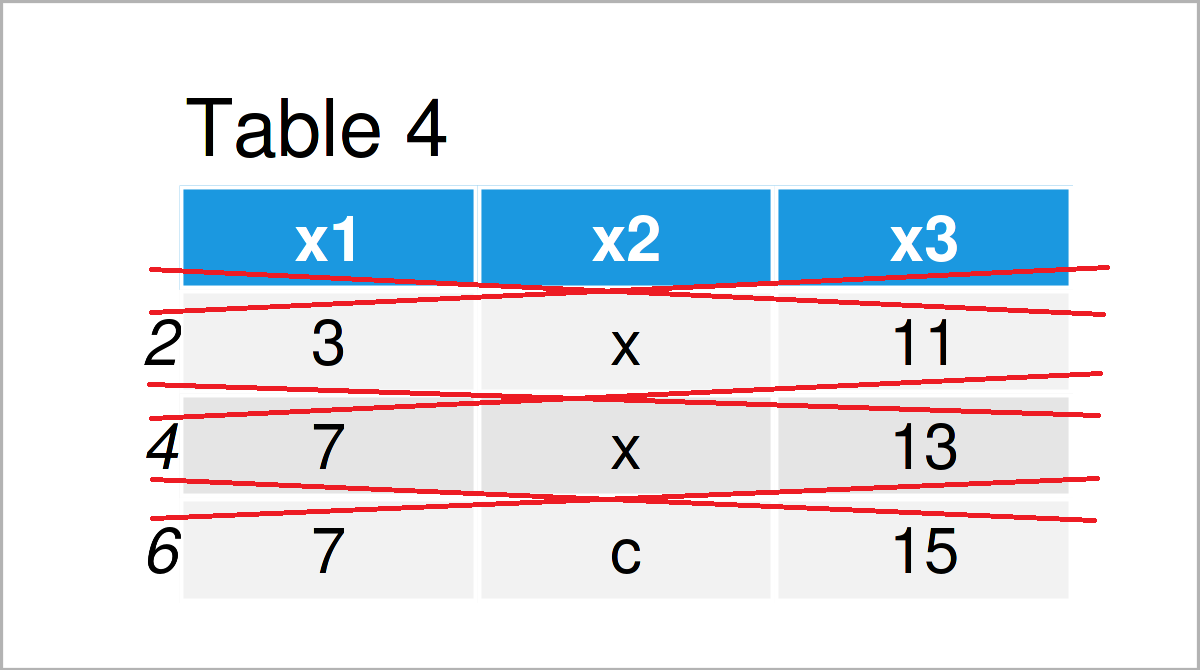As shown in Table 4, we have created another data frame where the rows No. 1, 3, and 4 have been dropped.

## Example 6: Add New Columns to Data Frame

In this section, I’ll demonstrate how to expand data frames in R.

More precisely, we’ll add a new column to our data frame.

For this, we have to create a vector object that we can append as a new variable later on:

```new_col <- 6:1                                  # Create vector object
new_col                                         # Print vector object
#  6 5 4 3 2 1```

As you can see, our vector object contains six numbers ranging from 6 to 1. Note that this vector needs to have the same length as the number of rows in our data frame.

Next, we can add this vector as a new column to our data matrix:

```data_new3 <- data                               # Create duplicate of data frame
data_new3\$x4 <- new_col                         # Add new column
data_new3                                       # Print updated data frame```The output of the previous R programming code is shown in Table 5: We have created another data frame containing the original data set plus an additional column called x4. This additional column contains the values of the vector object we have created before.

## Example 7: Replace Values in Data Frame

We may also exchange certain values in a data frame in R. In this section, I’ll show how to replace values in one column of a data frame based on a logical condition.

To achieve this, we can use the == operator as shown below:

```data_new4 <- data                               # Create duplicate of data frame
data_new4\$x1[data_new4\$x1 == 7] <- 555          # Replace values conditionally
data_new4                                       # Print updated data frame```In Table 6 you can see that we have managed to create another data frame where the value 7 in the column x1 was replaced by the value 999.

## Example 8: Export Data Frame to External File

The very last step when processing data frames in R, is often to export these data to an external file.

The following example code demonstrates how to save our manually created data frame as a CSV file using the write.csv2 function.

Consider the R code below:

`write.csv2(data, "data.csv") # Export data frame to CSV file`

A new CSV is created in our currently used working directory after executing the R syntax above: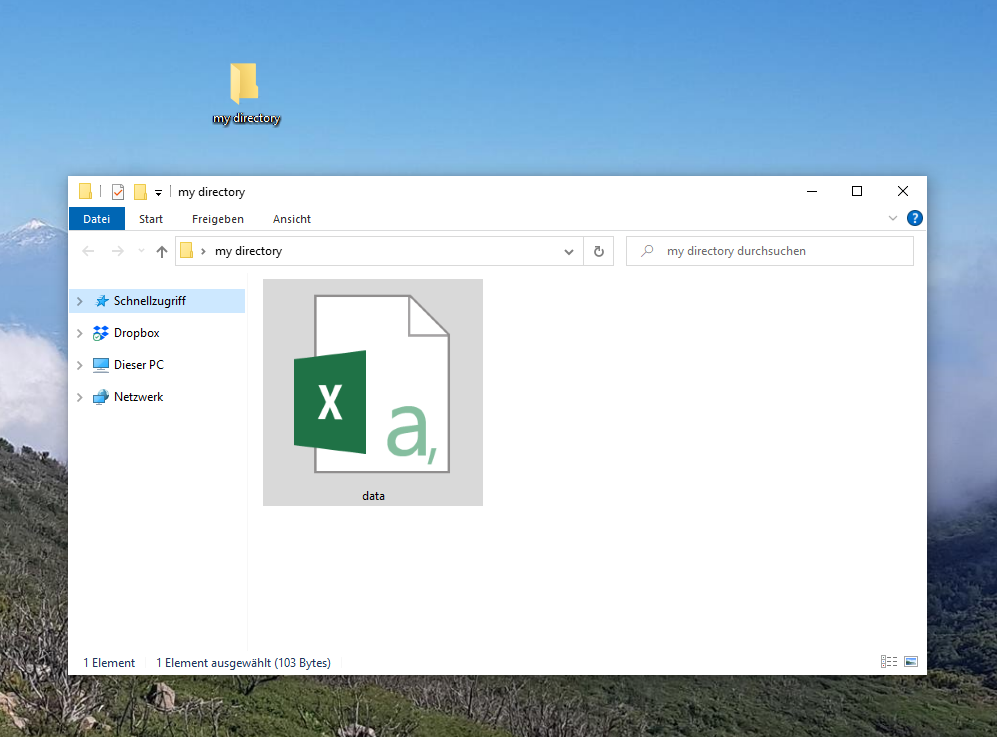We can see that this CSV file contains our data frame by opening it: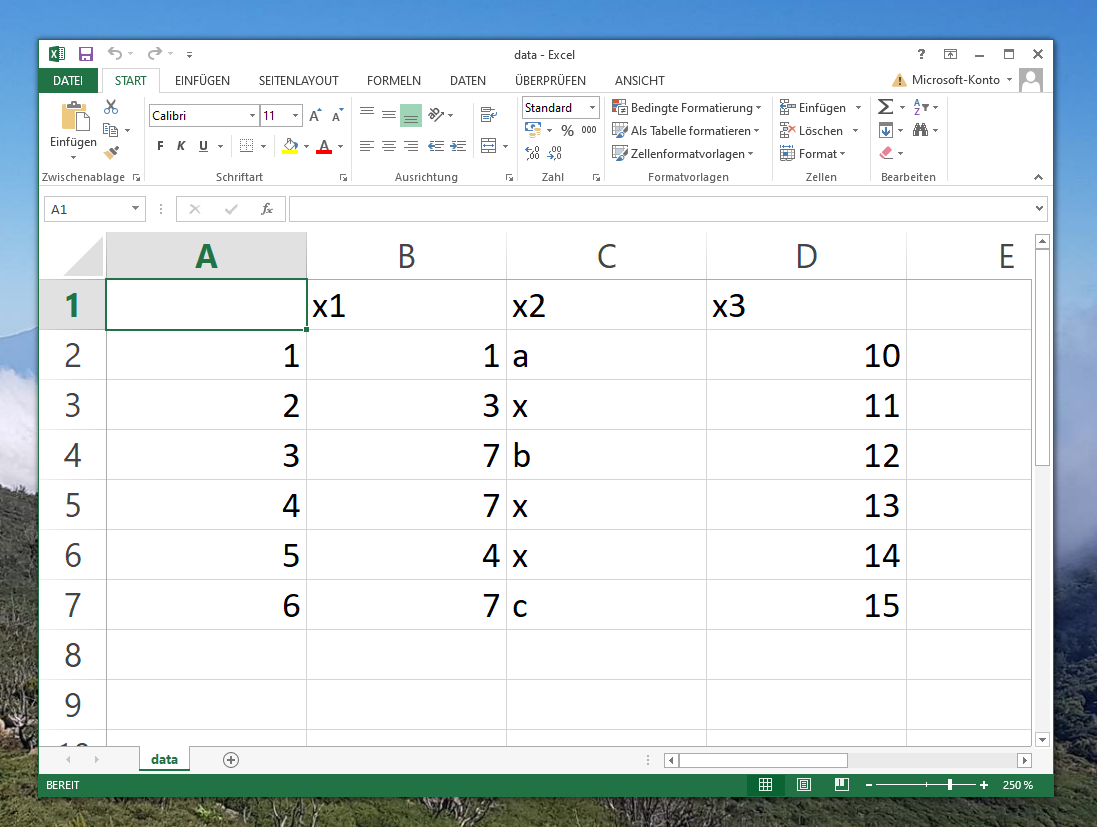Looks good! Now, we can use this file to distribute our data to other programmers and researchers, or we can use it to import our data back into R the next time we want to work on it.

## Video & Further Resources

Would you like to know more about the handling of data frames? Then you might watch the following video on my YouTube channel. In the video, I illustrate the examples of this article:

Besides the video, you could read the related tutorials which I have published on this homepage.

This post has demonstrated how to handle data frames in the R programming language. Don’t hesitate to please let me know in the comments section, if you have further questions.

Subscribe to the Statistics Globe Newsletter